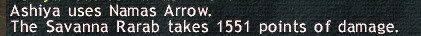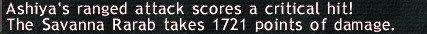# Yoichinoyumi Test #1.

1st MythYoichinoyumi has no DMG cap:

When people talk about DMG cap, they usually mean fSTR2 cap. You cannot change the maximum Weapon’s damage + Ammo damage, as well as the maximum pDiF (3 non-critical; 3.75 critical). So only fSTR2 is the variable factor when everything is capped in the damage formula:

Damage = floor((WD + AD + fSTR2) x pDiF)

Test on Low level monsters in West Sarutabaruta, which means pDiF is capped at 3. For the test of DMG cap, I used Yoichinoyumi + Kabura Arrows, which give Weapon’s damage + Ammo damage = 81 + 38 = 119

If fSTR2 is capped, it should be : fSTR2 = (Weapon’s rank + 8 ) x 2 = (floor(81/9) + 8 ) x 2 = 34

That means:

• Normal shot should be: (119+34) x 3 = 459
• Normal shot with piercing bonus 25%: (119+34) x 3 x 1.25 = 573Results from the test agree with the preliminary assumptions, which means Yoichinoyumi does have an fSTR2 cap.

Myth busted!

2nd Myth – Namas Arrow has WSC: 40% AGI, 40% STR; fTP = 2.75

In WS gears for the test, I have 96 STR (was /BLM >.>) and 112 AGI in total:

WSC = floor(floor((0.4×112+0.4×96)x0.83)) = 68

Damage = floor(floor((WD + AD + fSTR2 + 68) x 2.75) x 3)

Plug-in the number: For this test, I used Yoichinoyumi + Wooden Arrows, so total WD + AD = 81 + 5 = 86; fSTR2 should be capped from above test, so fSTR2 = 34; thus Namas damage should be:

Namas = floor(floor((86 + 34 + 68) x 2.75) x 3) = 1551Results from the 2nd test agree with this preliminary assumptions.

Myth confirmed!

Noted that the result was correct with the main assumption of fTP to be 2.75; if fTP is different, then theoretically, the WSC on Namas Arrow could be different, but since the result is exact to the last digit, I believe both fTP and WSC of Namas in the assumption are correct.

————————————————————-

An extra: This is the highest single shot damage that you can ever get with Yoichinoyumi, w/o PPA (or piercing bonus) :D### 11 Responses to “Yoichinoyumi Test #1.”

1.VZX says:

Although the result is the same, I think it’s good to point out small error in one of your calculation:
Namas = floor(floor((86 + 34 + 68) x 2.75) x 3) = 1551

So after multiplied by fTP, the number must also be integer. This way we categorize the number multiplied by fTP as “base damage”, still.

If you’re using kabura, then the number should be 1821

2.Ashiya says:

Thanks! Corretion incoming.

I got 1721 as the following:

Damage = floor((81 + 38 + 34) x 3) x 3.75) = 1721

I think it is the correct damage :/ Did I miss anything again?

3.VZX says:

Hmm yeah, a bit
you must floor on:
– after the base damage is tripled by hidden effect
– after multiplying by pDIF
– after multiplying by crit hit constant (1.25)

So:
Damage = floor(floor(floor((81 + 38 + 34) x 3) x 3.000)x1.25) = 1721

The number is right, it’s just a tiny bit detail on the exact calculation.

4.Ashiya says:

That is a lot of truncations XD Thanks for pointing it out VZX XD

5.Maiev says:

crazy stuff… crazy…

6.Jkun says:

So much maths. When did this stop being a game?

Oh right, as soon as I hit 75. Oh ho.

7.Ashiya says:

When PUP can’t figure out why they hit less than their pet!

8.Raiyoh says:

What does the floor variable stand for in the calculation?

9.Ashiya says:

It means you only take the integer part of the whole number – e.g. 3.5 will be known by the calculation as 3 only.

10.cyphx says:

i am working on getting a relic bow complete right now just some questions like one what would be perfect set up gear wise i am elvaan and two anyone ever seen SAM with soboro and yoichinoyumi go to work. Sorry if questions seem noobish but i am now getting back into rng and ls and friends helping me fund relic so i been surfing the net looking for best possible advice any give would be much appreciated.

11.Ashiya says:

I will try to post a set up of my gears soon. I need to make some free time to get it done though.